Courses

# Pure Substances - MCQ Test 1

## 20 Questions MCQ Test Mock Test Series - Mechanical Engineering (ME) for GATE 2020 | Pure Substances - MCQ Test 1

Description
This mock test of Pure Substances - MCQ Test 1 for Mechanical Engineering helps you for every Mechanical Engineering entrance exam. This contains 20 Multiple Choice Questions for Mechanical Engineering Pure Substances - MCQ Test 1 (mcq) to study with solutions a complete question bank. The solved questions answers in this Pure Substances - MCQ Test 1 quiz give you a good mix of easy questions and tough questions. Mechanical Engineering students definitely take this Pure Substances - MCQ Test 1 exercise for a better result in the exam. You can find other Pure Substances - MCQ Test 1 extra questions, long questions & short questions for Mechanical Engineering on EduRev as well by searching above.
QUESTION: 1

### In the figure shown, the system is a pure substance kept in a piston-cylinder arrangement. The system is initially a two-phase mixture containing 1 kg of liquid and 0.03 kg of vapour at a pressure of 100 kPa. Initially, the piston rests on a set of stops, as shown in the figure. A pressure of 200 kPa is required to exactly balance the weight of the piston and the outside atmospheric pressure. Heat transfer takes place into the system until its volume increases by 50%. Heat transfer to the system occurs in such a manner that the piston, when allowed to move, does so in a very slow (quasi-static I quasi-equilibrium) process. The thermal reservoir from which heat is transferred to the system has a temperature of 400°C. Average temperature of the system boundary can be taken as 17°C. The heat transfer to the system is I kJ, during which its entropy increases by 10 J/K. Atmospheric pressure.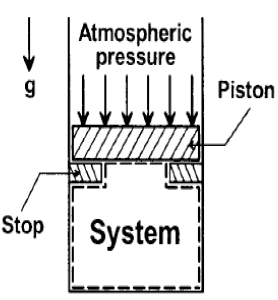Specific volumes of liquid (vf) and vapour (vg) phases, as well as values of saturation temperatures, are given in the table below.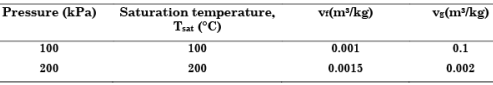At the end of the process, which one of the following situations will be true?

Solution:

Initial Volume (V1) = 0.001 + 0.03 X0.1 m3 = 0.004 m3

Let dryness fraction = x

Therefore 0.004 X 1.5 = (1 – x) X 0.0015 X 1.03 + x X 0.002 X 1.03

That gives an absurd value of x = 8.65 (It must be less than equal to unity). So vapour is superheated.

QUESTION: 2

### In the figure shown, the system is a pure substance kept in a piston-cylinder arrangement. The system is initially a two-phase mixture containing 1 kg of liquid and 0.03 kg of vapour at a pressure of 100 kPa. Initially, the piston rests on a set of stops, as shown in the figure. A pressure of 200 kPa is required to exactly balance the weight of the piston and the outside atmospheric pressure. Heat transfer takes place into the system until its volume increases by 50%. Heat transfer to the system occurs in such a manner that the piston, when allowed to move, does so in a very slow (quasi-static I quasi-equilibrium) process. The thermal reservoir from which heat is transferred to the system has a temperature of 400°C. Average temperature of the system boundary can be taken as 17°C. The heat transfer to the system is I kJ, during which its entropy increases by 10 J/K. Atmospheric pressure.Specific volumes of liquid (vf) and vapour (vg) phases, as well as values of saturation temperatures, are given in the table below.The net entropy generation (considering the system and the thermal reservoir together) during the process is closest to:

Solution: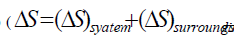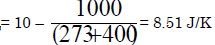QUESTION: 3

### A thermodynamic cycle with an ideal gas as working fluid is shown below.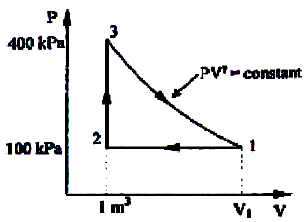If the specific heats of the working fluid are constant and the value of specific heat ratio γ is 1.4, the thermal efficiency (%) of the cycle is:

Solution: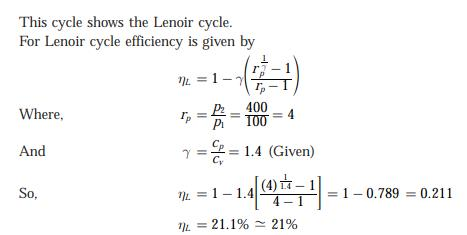QUESTION: 4

Constant pressure lines in the superheated region of the Mollier diagram have what type of slope?

Solution:

Mollier diagram is a h-s plot.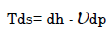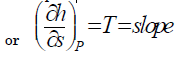T is always + ive so slope always +ive. Not only this if T Î then slope Î

QUESTION: 5

The following table of properties was printed out for saturated liquid and saturated vapour of ammonia. The titles for only the first two columns are available. All that we know is that the other columns (columns 3 to 8) contain data on specific properties, namely, internal energy (kJ/kg), enthalpy (kJ/kg) and entropy (kJ/kgK)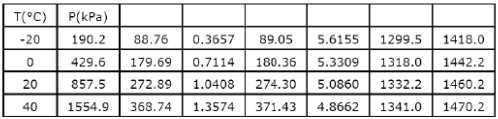The specific enthalpy data are in columns

Solution:
QUESTION: 6

When wet steam flows through a throttle valve and remains wet at exit

Solution:
QUESTION: 7

One kilomole of an ideal gas is throttled from an initial pressure of 0.5 MPa to 0.1 MPa. The initial temperature is 300 K. The entropy change of the universe is:

Solution: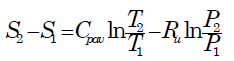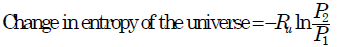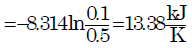QUESTION: 8

The given diagram shows an isometric cooling process 1–2 of a pure substance. The ordinate and abscissa are respectively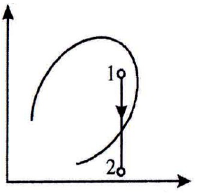Solution:
QUESTION: 9

The given diagram shows the throttling process of a pure substance.
The ordinate and abscissa are respectively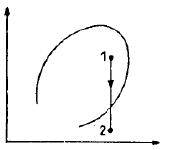Solution:

The throttling process given in figure is on pressure-enthalpy diagram.

QUESTION: 10

A piston –cylinder device contains 0.06m3 of saturated water vapour at 350 kPa pressure. Determine the temperature and mass of the vapour inside the cylinder.

Solution:

T = Tsat@350kPa = 138.86°C
v = vg@350kPa = 0.52422 m3/kg
m = V/v = 0.06 m3/0.52422 m3/kg = 0.114 kg.

QUESTION: 11

Assertion (A): At a given temperature, the enthalpy of super-heated steam is the same as that of saturated steam.

Reason (R): The enthalpy of vapour at lower pressures is dependent on temperature alone.

Solution:
QUESTION: 12

Which one of the following is correct?

The specific volume of water when heated from 0°C

Solution:

The largest density of water near atmospheric pressure is at 4°c.

QUESTION: 13

No substance can exist in the liquid phase in stable equilibrium

Solution:
QUESTION: 14

Consider the following statements about critical point of water:

1. The latent heat is zero.

2. The liquid is denser than its vapour.

3. Steam generators can operate above this point.

Of these statements

Solution:

At critical point, the latent heat in zero and steam generators can operate above this point as in the case of once through boilers.
The density of liquid and its vapour is however same and thus statement 2 is wrong.

QUESTION: 15

With increase of pressure, the latent heat of steam

Solution:
QUESTION: 16

Saturated liquid at a high pressure P1 having enthalpy of saturated liquid 1000 kJ/kg is throttled to a lower pressure P2. At pressure p2 enthalpy of saturated liquid and that of the saturated vapour are 800 and 2800 kJ/kg respectively. The dryness fraction of vapour after throttling process is:

Solution: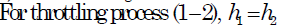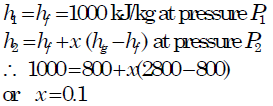QUESTION: 17

In a throttling process, which one of the following parameters remains constant?

Solution:

Consider a throttling process (also referred to as wire drawing process)

There is no work done (rising a weight) W = 0
If there is no heat transfer Q = 0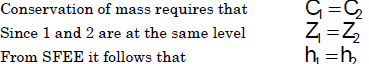Conclusion: Throttling is a constant enthalpy process (isenthalpic process)

QUESTION: 18

The process 1-2 for steam shown in the given figure is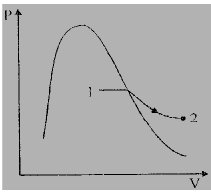Solution:
QUESTION: 19

The throttling process undergone by a gas across an orifice is shown by its states in the following figure: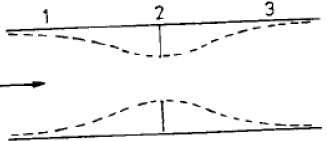Solution:

The throttling process takes places with enthalpy remaining constant. This process on T–S diagram is represented by a line starting diagonally from top to bottom.

QUESTION: 20

Assertion (A): On the enthalpy-entropy diagram of a pure substance the constant dryness fraction lines start from the critical point.

Reason (R): All the three phases co-exist at the critical point.

Solution:

Only two phase liquid-vapour is co-exists at the critical point, but at triple point-all three phase are co-exists.